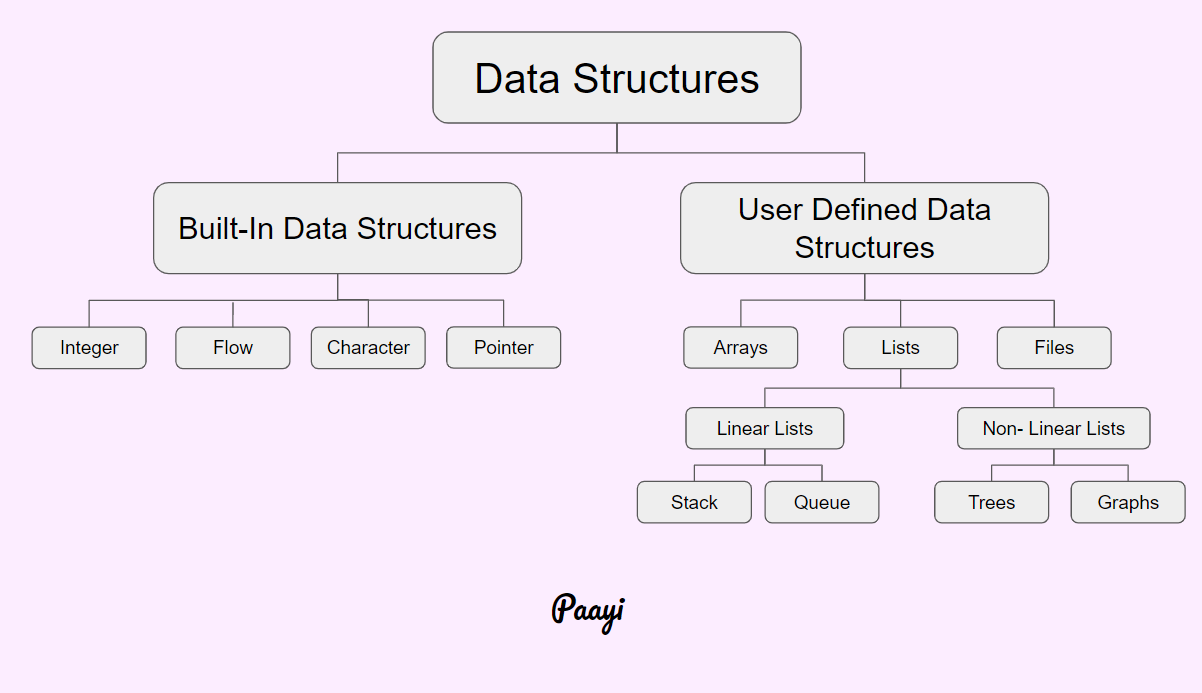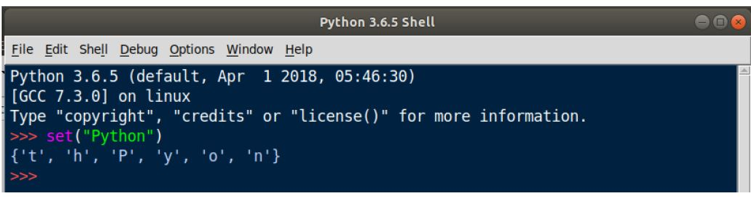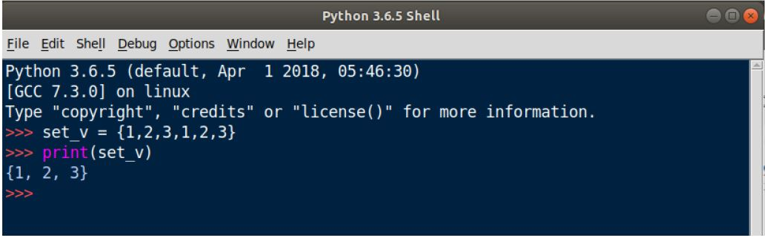## Python Tutorials: How to use Data Structures in Python

##### Python Tutorials: Learn Python Data Structures in Detail with Real Examples
Written by |17-Oct-2020 | 0 Comments | 1668 Views

In computer science, the data structure is one of the most important phenomena. It has more importance than classes. The data structure is a way of organizing the data in a proper format, which makes it easy to access and to modify the data at the time of need.

More precisely, we can say data structure is a way of organizing data and making a relationship between them.

There are lots of data structures, but basic data structures are divided into two categories. The first one is linear data structures, and the second one is the nonlinear data structure.

The linear data structures contain:

• Arrays
• Stack
• Queues

Also, nonlinear contains:

• Tree
• GraphIn python, there is no built-in array data structure; alternatively, we can use a list for that Function. However, at a higher level, we can see how to use arrays in python.

In addition to the data mentioned above, structure python provides other data structures, too, like sets and tuples.

Today we see how to use sets and tuples in python.

Sets:

Set is a type of data structure in python. It is similar to the mathematics set. There is no repetition of an element in a set. Unlike lists and arrays where the elements are stored in order, there is no order in sets.

The position of sets element is random. We can apply all the functionality of the set as we have studied in math in python too. We can add or remove the element from the set. We can apply union, intersection, etc. in sets. Any immutable can be inserted into the sets. For example, we can set a tuple as an element, but the list cannot be inserted into a set.

How to define Sets:

In python, sets can be defined by the set () function or by substituting the values within the curly braces. The following are the method to initiate the sets in python.

 Method 1:`>> set("Python")` Method 2:`>> set_variable = {"p","y","t","h","o","n"}`

Order of Set:

The order of an element is not essential in sets. The elements positions are changed when the sets are executed as follows:In figure 2 above, the order of an element is changed, as we can see.

Non-Repetition of Elements:

Inset, no element is repeated. The repeated element is removed from the set. Figure 3 shows this phenomenon:Inset, we can add elements by calling the add function. The following method is used to add the element.

 Code set1 = {1,2,3,4} set1.add (5)   print(set1)   Output {1,2,3,4,5}

Removing an Element:

To remove elements, there are two methods one is by calling the remove function, and the other is by calling the discard function. Both take one argument, and that is the value of the element that is to be removed. The following code can be used to remove elements.

 Code set1 = {1,2,3,4,5,6,7}   set1.remove(7) print(set1)   set1.discard(6) print(set1)   set1.pop () print(set1)   Output {1, 2, 3, 4, 5, 6} {1, 2, 3, 4, 5} {2, 3, 4, 5}

Operation on Set:

• Union Operation:
Union operation is done to merge all elements of two sets and combined to make it one set. Following is the method of union operation in python:

 Code a = {1,2,3,4,5} b = {6,7,8} print(a|b)   Output: {1,2,3,4,5,6,7,8}

• Intersection Operation:
It returns the common element of both set “A” and B. Following is the code of intersection:

 Code a = {1,2,3,4,5} b = {5,6,7,8} print(a&b) Output {5}

• Symmetric Difference:
It returns the element that either belongs to A or B but not from both. The following code can be used:

 Code a = {1,2,3,4,5,6} b = {1,2,3,4,5,7}   print(a^b)   Output {6,7}

• Subset:
To check if set A is a subset of set B, it can be verified by (A <= B). It returns true if it is subset otherwise false
• Superset:

To check if A is a superset of B, it can be verified by (A >= B). It returns true if it is superset; otherwise, it returns false.

Tuple:

A tuple is another type of data structure. It is similar to the list, but it is immutable. By immutable, we mean that tuple cannot be changed. Once the values are assigned to the tuple, no more value can be added or deleted from the tuple. Tuple uses parentheses, whereas, in the list, square brackets are used.

Tuples are an essential data structure. Mostly it is used where the data should not be changed just like a constant variable.

How to initialize tuple:

Tuple can be created by two methods one is by the keyword method, and the second one is by initializing the variable. The following methods are used to create a tuple.

 Code tuple1 = (1,2,3,4,5) tuple2 = tuple("Python")   print(tuple1) print(tuple2)
 Output (1, 2, 3, 4, 5) ('P', 'y', 't', 'h', 'o', 'n')

Assigning the value of a variable in a tuple:

We can assign multiple variables in one line by using the tuple. This allows the code to be more compact and written in less line of code, which ultimately makes the code cleaner. Following is the example of creating multiple variables in one line as follows:

 ` `Code string1, integer1, float1 = ("Python", 100, 3.47) print (string1) print (integer1) print (float1) Output Python 100 3.47

Comma Separated Value:

If the variable is initiated without parentheses separated by coma’s, python converts it into tuples. This tuple can be used then. The above example can be modified without parentheses as follows:

 Code a = "Python",100,3.47 string1, integer1, float1 = a print (string1) print (integer1) print (float1)
 Output Python 100 3.47

As we can see, there is no difference in the output.

Changing in the tuple:

As we discussed earlier, tuple values are immutable; they are not changeable. If we try to change or update, the value of the tuple python compiler can generate an error.

 tuple1 = ("Python",100,3.47)   tuple1 = "Programming"   print(tuple1)
 Output   Traceback (most recent call last):   File "/home/lalatoofani/PythonProgramming.py", line 3, in     tuple1 = "Programming" TypeError: 'tuple' object does not support item assignment

As the error indicates, tuple doesn’t allow any changes which make it immutable.

As we saw earlier that we could not change the tuple and its value. This is valid only for the data types like string, integer, or float, but what happens if a tuple contains mutable data type as a list in it. In such a scenario value of the list can be changed within a tuple. Let us take a look at how we can change the value of mutable data type within a no mutable tuple:

 ` `Code tuple1 = ("Python",100,3.47, [1,2,3,4,5,6]) tuple1 ="New Value" print(tuple1)

In the above code, we first initialize a tuple that has 4 values. At index 3, we have a list that contains multiple values. Now by accessing the 3rd index and then accessing its 2nd index, we change the value of the list from three to new values. The program can be run to check its output:

 Output ('Python', 100, 3.47, [1, 2, 'New Value', 4, 5, 6])

As we can see from the above line, the value has been changed from 3 to New Value.

Built-in Function of tuples:

1. max (): max Function returns the maximum value of the tuple
2. min (): min function returns the minimum value of the tuple
3. Len (): len function returns the length of a tuple.
4. tuple (): tuple function converts any list or string into tuples.
5. sorted (): returns the sorted tuple

Checking Existence:

Generally, in other languages, to check if the value is present in a data structure or not, we have to iterate to all of the value and match from our desired value. However, no in the case of python. Python allows the user to check value existence in just one line as follows:

 Code tuple1 = ("Python",100,3.47, [1,2,3,4,5,6]) print ("Python" in tuple1) print (100 not in tuple1)

The output of the above program is True and then False as “Python” is in the tuple, and the second statement we say 100 not present in the tuple, which is false as 100 is in the tuple.

Accessing tuple:

The following methods can access the value of tuple:

1. Looping
2. Indexing
3. Negative Indexing

Looping:

In the looping technique, we loop over each element of the tuple to get the value. The following are the ways to loop over the tuple:

 Code tuple1 = ("Python",100,3.47, [1,2,3,4,5,6]) for e in tuple1: print(e) for i in range(len(tuple1)):  print(tuple1[i])

Both these loops can perform the same action.

Indexing:

By the method of indexing, we have to provide the index to access the value. The following are the method of indexing:

` `Code

 tuple1 = ("Python",100,3.47, [1,2,3,4,5,6]) Output 3.47 print(tuple1) Output ("Python",100,3.47) print(tuple1[0:3])

Negative Indexing:

Negative indexing is the opposite of indexing. As indexing starts from the start, negative indexing starts from last as follows:

Code

 tuple1 = ("Python",100,3.47, [1,2,3,4,5,6]) Output [1,2,3,4,5,6] print(tuple1[-1])